The XY model and the Ising model in one and two dimensions¶

Examples - Statistical Mechanics¶

Last edited: March 22nd 2018

Introduction¶

In this example we discuss the physics of the classical paramagnet and the Ising model in one and two dimensions, and along the way touch on general aspects of statistical mechanics.

The Ising model is a mathematical model in statistical mechanics, which can describe the phenomenon of ferromagnetism. The model consists of discrete variables that represent magnetic dipole moments of atomic $\textit{spins}$ that can be in one of two states (+1 or -1). The spins can be arranged in a lattice, allowing each spin to interact with its neighbours. The model allows for the identification of phase transitions, as a simplified model of reality. The two-dimensional square lattice Ising model is one of the simplest statistical models to show a phase transition .

The XY model is an important spin model of easy plane anisotropic magnetic insulators where spins $\mathbf{S}_i$ with two components $\mathbf{S}_i = \left(S_{xi}, S_{yi}, 0\right)$ reside on a set of lattice points . (It is also a much used effective description of superfluids such as He$^{4}$ at low temperatures, which is a condensed matter system with dissipation-less transport of matter! ) Here, we take the lattice to be a three-dimensional cubic lattice. The Hamiltonian of this spin system is given by

\begin{align*} H = -J \sum \limits_{\left< i,j \right>} \mathbf{S_i} \cdot \mathbf{S_j} - \mathbf{h} \cdot \sum\limits_{i=1}^{N} \mathbf{S_i}, \end{align*}

where $\left< i, j \right>$ means that lattice sites $i$ and $j$ are nearest neighbours, and $J$ is the energy that couples together spins on nearest neighbour lattice sites. The vector $\textbf{h}$ represents an external magnetic field.

Analytical solution of the XY paramagnet¶

Consider a collection of $N$ independent XY spins $\mathbf{S}_i = \left(S_{xi}, S_{yi}, 0\right)$ in an external magnetic field $\mathbf{h} = \left(h, 0, 0\right)$, such that $\mathbf{h} \cdot \mathbf{S}_i = hS\cos(\phi_i)$, where $\phi_i$ is the angle between the spin and the external magnetic field, and $S$ is the length of the spin, which we consider to be fixed. We set $J$ = 0, such that all spins are independent. The Hamiltonian of this system is

\begin{align*} H = - \mathbf{h} \cdot \sum\limits_{i=1}^{N} \mathbf{S_i} = -hS \sum\limits_{i=1}^{N} \cos(\phi_i), \end{align*}

with the system illustrated below.Canonical partition function $Z$¶

To determine thermodynamic quantities such as the pressure, energy density and the entropy of the system, we calculate the partition function $Z$, in our case the classical canonical one, given in general as

\begin{align*} Z = \sum\limits_{i=1} e^{-\beta E_i}, \end{align*}

where $\beta$ is a so-called Lagrange multiplier, and can be found to be equal to $1/k_B T$. (It arises from our goal of finding the most probable energy distribution subject to certain constraints. Further details can be found in any standard textbook on statistical mechanics, e.g. .) The partition function $Z$ is one of the most important quantities in equilibrium statistical mechanics. It is a sum over all microstates $i$. In quantum mechanics, the index $i$ is typically a discrete variable and the partition function is a dimensionless variable. In classical mechanics, the variable $i$ often becomes continuous and the sum is replaced by an integral. In our case $\phi \in [0, 2\pi)$ is a continuous variable, thus inserting our expression for $H$ into our expression for $Z$, we get

\begin{align*} Z &= \sum\limits_{\{\mathbf{S_i}\}} e^{-\beta H} = (Z_1)^N \\ Z_1 &= \sum\limits_{\mathbf{S}} e^{\beta \mathbf{h} \cdot \mathbf{S}} = \int_0^{2\pi} e^{\beta h S \cos\phi} d\phi= 2\pi I_0(\beta h), \end{align*}

where $I_0$ is the modified Bessel function of the first kind and order zero. In the last step, we have set $S = 1$ for convenience. See the appendix for more details about the modified Bessel function. We plot the modified Bessel function of orders 0 through 3, to aid our intuition. Codewise, we import the necessary packages and set common figure parameters. We use the implementation of the modified Bessel function provided in the scipy library, scipy.special.i0 for order 0 and scipy.special.iv for higher orders $v$.

In :
import numpy as np
import matplotlib.pyplot as plt
import scipy.special as sci
import time
import itertools as it
from matplotlib import rc
plt.style.use('bmh')
In :
# --- Set common figure parameters
newparams = {'font.size': 14}
plt.rcParams.update(newparams)
In :
plt.figure(figsize=(16, 4))
x = np.linspace(0, 4, 100)
plt.plot(x, sci.i0(x));
plt.plot(x, sci.iv(1, x));
plt.plot(x, sci.iv(2, x));
plt.plot(x, sci.iv(3, x));
plt.title('Modified Bessel function')
plt.xlabel('$x$')
plt.ylabel('$I_v$')
plt.legend(['$I_0$', '$I_1$', '$I_2$', '$I_3$'], loc=2)
plt.show()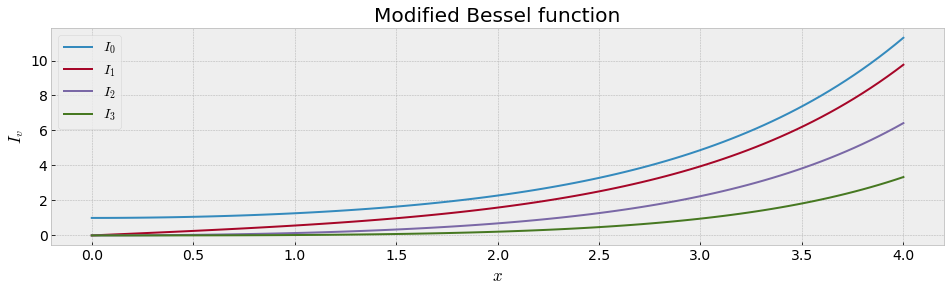Average magnetisation per spin $m(h, T)$¶

Now that we know $Z$, we decide to calculate the component $M$ of the average magnetisation that is parallel to the external field and the specific heat $C_h$ (at constant magnetic field) of our system. Let $m(h, T)$ denote the average magnetisation per spin, which is defined by

\begin{align*} m &\equiv \frac{1}{N} \left<\hat{h} \cdot \mathbf{M}\right> \\ &= \frac{1}{N} \left<\hat{h} \cdot \sum_i \mathbf{S}_i\right> \\ \left<\hat{h} \cdot \mathbf{M}\right> &= \frac{\partial(\ln{Z})}{\partial(\beta h)} = N \frac{I_0'(\beta h)}{I_0(\beta h)} = Nm \\ \Rightarrow m(h, T) &= \frac{I_1(\beta h)}{I_0(\beta h)}, \end{align*}

where we have used the relation $I_0' = I_1$. It is of particular interest to investigate the values of $m$ when $\beta h S \ll 1$. A plot is useful when we want to gain a bit of physical insight:

In :
plt.figure(figsize=(16, 4))
beta_hs = np.linspace(0, 16, 200)
plt.plot(beta_hs, sci.i1(beta_hs)/sci.i0(beta_hs));
plt.title('Magnetisation per spin in the XY model')
plt.xlabel('$\\beta h$')
plt.ylabel('$m$')
plt.show()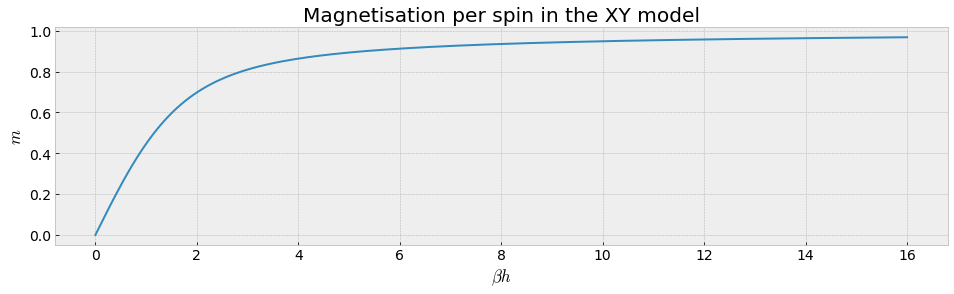We observe that $m \to 0$ as $\beta h \to 0$. This is the case when $h \to 0$ or $T \to \infty$. The first limit means that the average magnetisation vanishes as we remove the external field; all microstates have the same energy and thus also equal probabilities, which makes the average magnetisation go to zero. The latter limit means that as the temperature rises, the thermal fluctuations will begin to dominate the aligning effect of the external field.

The function $m(h, T)$ is analytic everywhere, including $(0,0)$, hence $\lim\!_{(h,T)\to(0,0)}\; m(h,T) = 0$ for any path we let $(h,T)$ approach the origin. In other words, it is not possible to retain a net magnetisation after the external field has been removed - just as one would expect when there is no coupling between the spins!

Specific heat per spin $C_h$¶

To calculate $C_h$, we first find the enthalpy $H_E = \langle H \rangle$ of our system

\begin{align*} H_E &= - \frac{\partial (\ln{Z})}{\partial \beta} = -h N \frac{I_1(\beta h)}{I_0(\beta h)} \\ C_h &= \frac{1}{N} \left(\frac{\partial H_E}{\partial T}\right)_h = -h \frac{\partial(I_1/I_0)}{\partial (\beta h)} \frac{\partial (\beta h)}{\partial T} \\ &= \left(\frac{-h^2}{-k_B T^2}\right) \cdot \left(\frac{I_1'}{I_0} - \frac{I_1^2}{I_0^2}\right) \\ &= k_B h^2 \frac{\beta^2}{I_0^2} (I_1'I_0 - I_1^2). \end{align*}

By using the recursion relations we find

\begin{align*} I_1' &= \frac{d}{dx}(xI_1) = I_1 + xI_1' = xI_0, \\ I_1' &= \frac{1}{x}(xI_0 - I_1), \\ I_1'I_0 - I_1^2 &= I_0(I_0 - \frac{I_1}{x}) - I_1^2, \\ x \rightarrow 0 : I_0 &\rightarrow 1, \\ x \ll 1 : I_0 &\simeq 1, \\ I_1 &\simeq \frac{x}{2}, \\ \implies C_h &\simeq \frac{1}{2} k_B (\beta h)^2. \end{align*}

What happens when $h \to 0$? We get that $C_h \to 0$! The reason for this is as follows: Another way of writing the specific heat is in terms of energy fluctuations

\begin{align*} C_h &= k_B \beta^2 \left[\langle H^2 \rangle - \langle H \rangle^2\right], \\ H &= - \mathbf{h} \cdot \sum\limits_{i=1}^N \mathbf{S}_i. \end{align*}

$h \to 0 \implies H \to 0 \implies$ no energy fluctuations $\implies$ no specific heat $C_h$.

Numerical solution of the Ising model in one and two dimensions¶

We now move from discussing the analytical solution of the paramagnet to the numerical solution of the Ising model in one and two dimensions. It is a simple, but very important model of $N$ interacting spins, which reside on points of some lattice. It can thus serve as a model of magnetic insulators, but has also a wide variety of applications in many other systems. Since many of its properties are known exactly in one and two dimensions (and with great precision also in three dimensions based on numerical work), it serves as a useful benchmark for approximation schemes and calculational schemes. In this problem, we will study the model numerically on a one-dimensional chain and on a two-dimensional square lattice with periodic boundary conditions. The model is defined by the Hamiltonian

\begin{align*} H = -J \sum\limits_{\left< i,j \right>} s_i s_j - h \sum\limits_i s_i, \end{align*}

where $\left< i,j \right>$ means that lattice sites $i$ and $j$ are nearest neighbours, $J > 0$ is the energy that couples together spins on nearest neighbour lattice sites, and $h$ is an external magnetic field. Without loss of generality, we set $h > 0$. Finally, $s_i$ are the spin variables of the system, which can take the values $s_i =\pm 1$.

The canonical partition function of this system is then given by

\begin{align*} Z = \sum\limits_{\{s_i\}} e^{-\beta H}. \end{align*}

This model may be studied with two different conditions on the boundary spins:

i) Open boundary conditions. Here, the spins on the edge of the lattice only interact with the spins in the interior of the lattice.

ii) Periodic boundary conditions. Here, the spins on the edge of the lattice interact with the spins in the interior of the lattice and with the spins on the same row or column on the opposite edge. (This corresponds to wrapping a two-dimensional square lattice into a torus).

In this example we use periodic boundary conditions.

Computing the quantities $Z$, $U$, $C_h$ and $m$ (per spin)¶

We want to compute numerically the partition function $Z$, the internal energy $U$, the specific heat $C_h$ and the average magnetisation $m$ for the one-dimensional lattice and square lattices with dimensions $2 \times 2$, $3 \times 3$, $4 \times 4$ as functions of temperature. Computations for the parameters $N=16$ and $h/J \in \{0, 1/2, 1\}$ are performed and then the thermodynamic quantities are plotted.

In :
def generate_nn_array(N, D):
"""Generate array of nearest neighbour (nn) indices.
D=1: This is pretty straightforward, spin i interacts with spin i+1 mod N
D=2: This is a bit trickier. Even though we have two dimensions, we
represent our grid as a one dimensional array (a vector). The grid may be
written as (for a 3x3 lattice, the number is the index of the array:

6 7 8
3 4 5
0 1 2

This means that we need an N x 2 array which contains the indices of the
nn in the positive x and y directions. For instance, nn[1, 0] should be
equal to 1, nn[0, 1] should be equal to 3.

:N: number of lattice sites in total (not linear size)
:D: lattice dimension (if D=2, make sure N is a perfect square)
:returns: nn array (number of sites, direction 0:+ 1:-)
"""
nns = np.zeros((N, D), dtype=int)
if D == 1:
nns[:, 0] = [(i+1)%N for i in range(N)]
elif D == 2:
stride = int(np.sqrt(N))
for i in range(N):
nns[i, 0] = (i+1)%stride + np.floor(i/stride)*stride
nns[i, 1] = (i+stride)%N
else:
print('D not supported')
return None
return nns

def hamiltonian(conf, J, h, nns, N, D):
"""Calculate the Hamiltonian for a given configuration by looping over each
lattice site and calculating s_i*s_j for each nearest neighbor, as well as
the external field contribution h.

:conf: array of a spin configuration
:returns: value of H
"""
H = 0.
for i in range(N):
for j in range(D):
# --- nn term in direction j
H += -J * conf[i] * conf[nns[i, j]]
# --- external field contribution
H += -h*conf[i]
return H

def compute_quantities(N=4, D=2, J=1., h=0.5, nt=201, ts=0.5, te=5.):
"""Compute the thermodynamic quantities.
:N: number of lattice sites
:D: number of dimensions (1 & 2 supported)
:J: coupling strength
:h: external magnetic field strength
:nt: number of temperature steps
:ts: start temperature
:te: end temperature
:returns: a tuple containing the thermodynamic quantities
(temps, lnZ, Ch, U, mag).
"""
nns = generate_nn_array(N, D)

# --- Initialize arrays for measurements
temps = np.linspace(ts, te, num=nt, endpoint=True)
lnZ = np.zeros(nt)
mag = np.zeros(nt)
U = np.zeros(nt)
Ch = np.zeros(nt)

# --- Start clock for timing
st = time.time()

# --- Create all possible configurations of +- spins on N sites.
# --- it.product is a function from the itertools package that creates an iterable
# --- (an object that can be looped over) which successively creates all possible
# --- combinations of +-1
configurations = it.product([1, -1], repeat=N)
# --- Loop over configurations
for c in configurations:
# --- Calculate the value of H
ham = hamiltonian(c, J, h, nns, N, D)
# --- Loop over temperatures
# for i, t in enumerate(temps):
# --- Calculate contribution to the partition function and observables
# --- from the given configuration and add to the appropriate arrays.
# --- Here we use vector operations, as it is more effective.
bman = np.exp(-ham/temps)
lnZ += bman
# --- <H>
U += ham*bman
# --- <H^2>
Ch += ham**2*bman
# --- <m>
mag += sum(c)*bman

runtime = (time.time() - st)/len(temps)
print('Average of {0:4.3f} s per temp iteration.'.format(runtime))

# --- Normalize results
mag /= (lnZ*N)
U /= lnZ
Ch = (Ch/lnZ-U**2)/temps**2/N
U /= N
lnZ = np.log(lnZ)

return (temps, lnZ, Ch, U, mag)

def generate_plots(N=4, D=2, nt=201, ts=0.5, te=5.):
"""Compute and plot the thermodynamic quantities for h/J = 0, 0.5 and 1.
"""
fig = plt.figure(figsize=(16, 10))
fig.suptitle('Parameters: D = {0}, N = {1}'.format(D, N), y=1.0)

J = 1.0
h_values = [0.0, 0.5, 1.0]

for h in h_values:
(temps, lnZ, Ch, U, mag) = compute_quantities(N, D, J, h, nt, ts, te)

ax1.plot(temps, lnZ)
ax1.set_ylabel(r'$\ln Z$')
ax1.set_xlabel(r'$k_B T$')

ax2.plot(temps, U)
ax2.set_ylabel(r'$U$')
ax2.set_xlabel(r'$k_B T$')

ax3.plot(temps, Ch)
ax3.set_ylabel(r'$C_h$')
ax3.set_xlabel(r'$k_B T$')

ax4.plot(temps, mag)
ax4.set_xlabel(r'$k_B T$')
ax4.set_ylabel(r'$\langle m \rangle$')

legend_info = ['h = {0}'.format(h) for h in h_values]
ax1.legend(legend_info)
ax2.legend(legend_info, loc='lower right')
ax3.legend(legend_info)
ax4.legend(legend_info)
fig.tight_layout()
In :
start_time = time.time()

generate_plots(N=16, D=1)
generate_plots(N=16, D=2)

print("Program runtime: %.5s s." % (time.time() - start_time))
Average of 0.010 s per temp iteration.
Average of 0.009 s per temp iteration.
Average of 0.009 s per temp iteration.
Average of 0.011 s per temp iteration.
Average of 0.011 s per temp iteration.
Average of 0.011 s per temp iteration.
Program runtime: 12.46 s.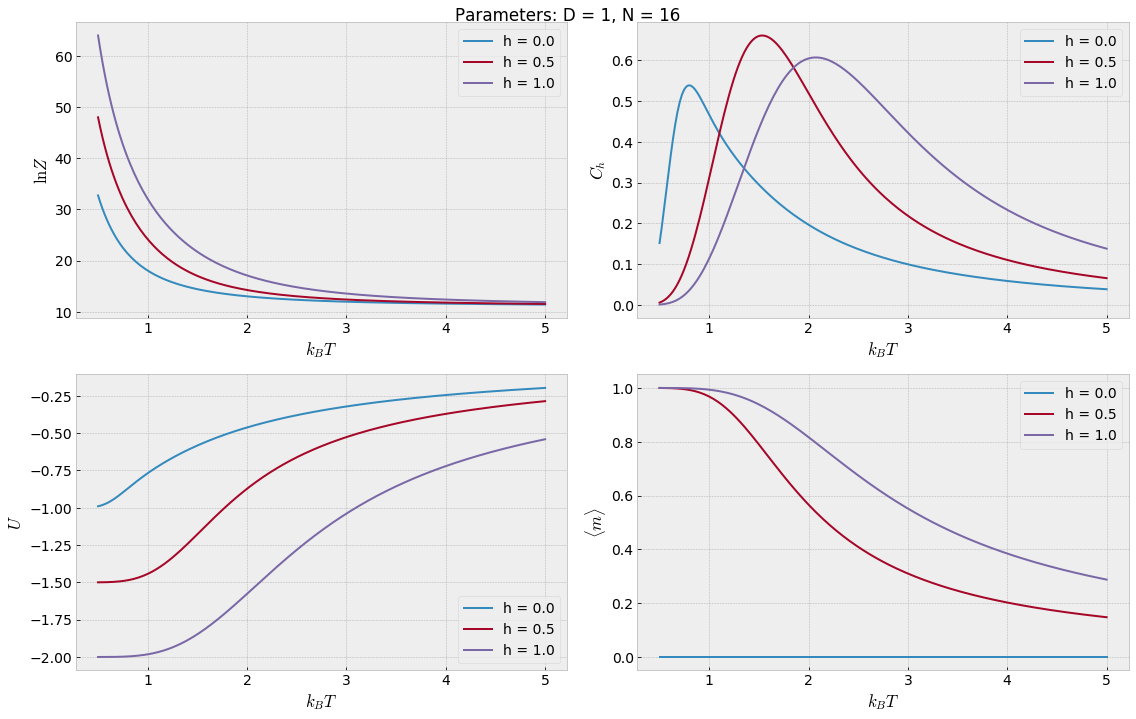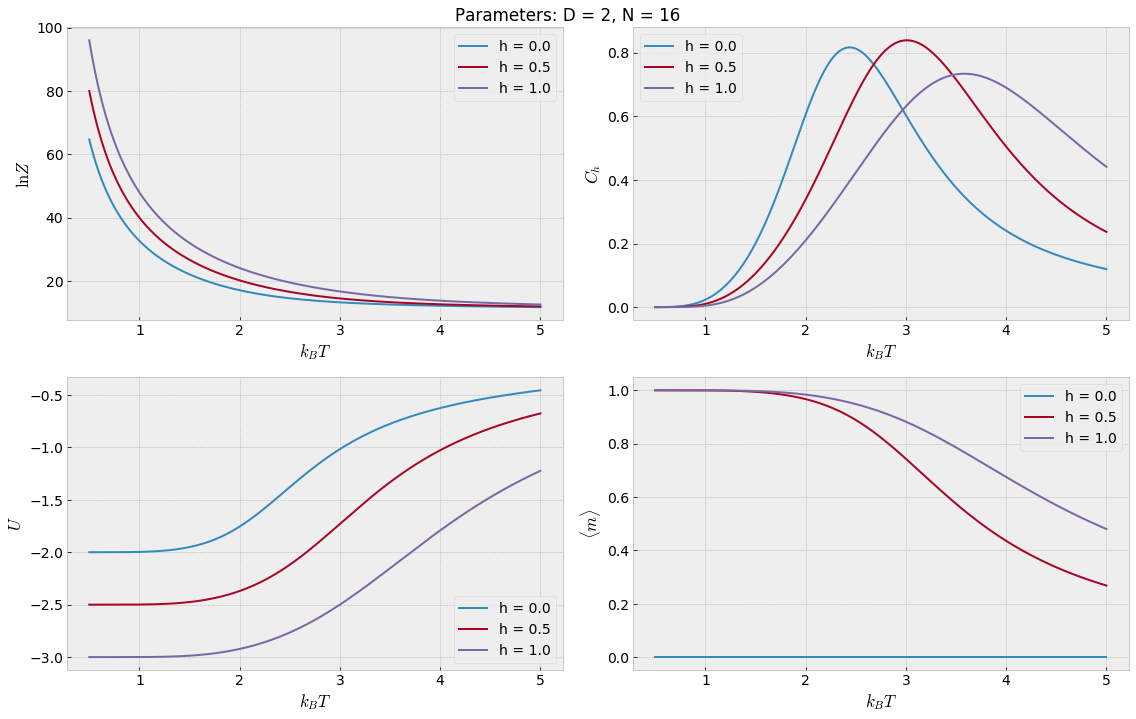The observant reader may have noticed that these values of $k_B T$ correspond to ridiculously high temperatures. There is no need to worry though, as in a more realistic situation, the Hamiltonian would typically be scaled by a factor with order of magnitude comparable to that of the Bohr magneton $\mu_B = 9.27 \times 10^{-24} \mathrm{J/T}$. We discover that the specific heat has a distinct peak at low temperatures that gets shifted to the right as $h$ increases. This type of peak is in general referred to as a Schottky anomaly . The anomaly occurs because of the energy gap between the ground state and the first excited state:

Let $\Delta E$ denote the size of this energy gap. At low temperatures $k_B T \ll \Delta E$, the thermal energy is not large enough to overcome the energy gap $\implies$ few energy states available $\implies$ small $C_h$. When $k_B T \sim \Delta E$, there is a sudden increase in the number of available energy states, hence a sudden increase in $C_h$. The specific heat will decline again as the temperature rises further due to the factor $1/k_B T^2$ and the finite number of energy states, resulting in the observed peak. When we increase $h$, we also increase the energy gap and the peak gets shifted towards higher temperatures. This explains the trend we observe in the plots.

The specific heat goes to zero in the extreme limits of the temperature. There is only one available energy state in the limit $T \to 0$, namely the ground state, which implies that there are no energy fluctuations and therefore $C_h = 0$. When $T$ approaches infinity, the spins will gradually become more randomly oriented, and the magnetisation and the internal energy will therefore approach zero. The rates at which $U$ and $m$ change with respect to $T$ also drop at higher temperatures, because the randomness of the spins becomes less sensitive to changes in $T$ as the temperature gets very high.
Another expression for the specific heat is $C_h = \frac{\partial \langle E \rangle}{\partial T}$, and we thus see that $C_h \to 0$ as $T \to \infty$. At first glance it might seem counterintuitive that the internal energy goes to zero as $T \to \infty$, but remember that we do not take into account any forms of kinetic energy.

The specific heat $C_h$ in the 1D and 2D model¶

Furthermore, we let $h = 0$ and investigate the computed values of $C_h$ in both the one-dimensional and the two-dimensional case for $N \in \{4, 9, 16\}$.

In :
start_time = time.time()

fig = plt.figure(figsize=(10, 5))
N_values = [4, 9, 16]

for N in N_values:
(temps, lnZ, Ch, U, mag) = compute_quantities(N, D=1, J=1.0, h=0, ts=0.1, te=6.0)
plt.plot(temps, Ch, '--')
(temps, lnZ, Ch, U, mag) = compute_quantities(N, D=2, J=1.0, h=0, ts=0.1, te=6.0)
plt.plot(temps, Ch)

plt.xlabel('$k_B T$')
plt.ylabel('$C_h$')
plt.title('Specific heat')
legend_info = ['N = {0}, D = {1}'.format(N, D) for N in N_values for D in [1, 2]]
plt.legend(legend_info)
print("Program runtime: %.5s s." % (time.time() - start_time))
Average of 0.000 s per temp iteration.
Average of 0.000 s per temp iteration.
Average of 0.000 s per temp iteration.
Average of 0.000 s per temp iteration.
Average of 0.009 s per temp iteration.
Average of 0.011 s per temp iteration.
Program runtime: 3.900 s.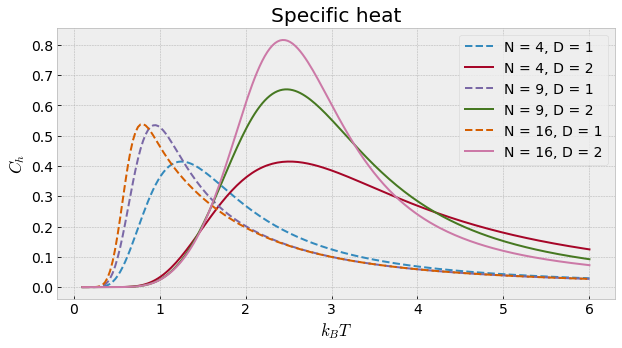The peaks in the 2D model occur at higher temperatures than in the 1D model. This can be explained as follows: There are more nearest neighbour spins in the 2D model, which means that it takes more energy to flip a spin, increasing the energy gap between the ground state and the first excited state.

As a final note, we recommend that you use a compiled language for computations involving larger numbers of spins $N$ (provided that you are going to stick with this method), as the calculations become more computationally demanding. Solutions for both the 1D and the 2D Ising model implemented in Fortran can be found here:

Another approach would be the use of Monte Carlo methods, which is certainly more feasible for larger lattices.

 Wikipedia Ising model (Acquired April 20th 2016)

 Homework 3 TFY4230 Statistical Physics, Spring 2016. http://web.phys.ntnu.no/~asudbo/TFY4230_2016/Oving3.pdf (Acquired March 1st 2016)

 Youtube Ben Miller experiments with superfluid helium - Horizon: What is One Degree? - BBC Two (Visited April 20th 2016)

 Jens O. Andersen, Introduction to Statistical Mechanics, 1st Edition, 2012, Akademika Forlag.

 R. K. Pathria & P. D. Beale, Statistical Mechanics, 3rd Edition, 2011, Butterworth–Heinemann, p. 79.

Appendix¶

Modified Bessel function¶

We have the following recursion relation between modified Bessel functions of the first kind and order $n$, $I_n(x)$

\begin{align*} x\frac{dI_n(x)}{dx} + nI_n(x) &= xI_{n-1}(x) \\ \frac{dI_0(x)}{dx} &= I_1(x). \end{align*}

Furthermore, we have the series representations of $I_0(x)$ and $I_1(x)$

\begin{align*} I_0(x) &= \sum\limits_{k=0}^{\infty} \frac{\left(\frac{x}{2}\right)^{2k}}{(k!)^2} \\ I_1(x) &= \sum\limits_{k=0}^{\infty} \frac{\left(\frac{x}{2}\right)^{2k+1}}{(k!)(k+1)!}. \end{align*}

Acknowledgements¶

This notebook was based on a numerical exercise in the course TFY4230 Statistical Mechanics at NTNU (2016).
Special thanks go to Prof. Asle Sudbø for letting us use his exercise and to PhD. student Peder N. Galteland for the Python code.